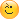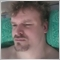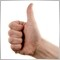Quality and efficiency of mql coding.39054

There was recently an interesting topic on Russian forum, where the discussion started from a poll on the quality of code produced by freelancer to finally shift to a discussion on the best way to retrieve the name of a timeframe (as s string) from its mql4 value.

The big divergence start from the following code, posted as a "proof" of bad quality code :

string TFtoString(int tf)
{
string TF="";
if(tf==0)tf=Period();
if(tf==1)TF="M1";
if(tf==5)TF="M5";
if(tf==15)TF="M15";
if(tf==30)TF="M30";
if(tf==60)TF="H1";
if(tf==240)TF="H4";
if(tf==1440)TF="D1";
if(tf==10080)TF="W1";
if(tf==43200)TF="MN1";
return(TF);
}

Unfortunately, as it's often the case on Russian forum, there was not any useful conclusion. So I decide to check by myself.

I created a script to benchmark all solutions I could find on this topic, on the forums in general, including my own solution. There was 8 proposed methods, one being discarded as not working, remains 7 methods. These methods are presented "as is", the author doesn't necessarily had efficiency in mind when posting the code.

The methods :

//+------------------------------------------------------------------+
//| Method 1 : using static arrays (no enums, no switch)             |
//+------------------------------------------------------------------+
string PeriodToString_StaticArrays(int tf)
{
static string tfLabel[]={"M1","M5","M15","M30","H1","H4","D1","W1","MN1"};
static int tfInt[]={PERIOD_M1,PERIOD_M5,PERIOD_M15,PERIOD_M30,PERIOD_H1,PERIOD_H4,PERIOD_D1,PERIOD_W1,PERIOD_MN1};
int index=-1;

//--- check for 0 : current
if(tf==0) tf=_Period;
//--- search index
for(int i=0;i<9;i++)
{
if(tfInt[i]==tf)
{
index=i;
break;
}
}
//--- check if found
if(index==-1)
{
return("Unknown timeframe");
}
//--- result
return(tfLabel[index]);
}
//+------------------------------------------------------------------+
//| Method x : using ternary operator                                |
//| https://www.mql5.com/en/docs/basis/operators/ternary             |
//| https://www.mql5.com/ru/forum/40117/page29#comment_1324723       |
//+------------------------------------------------------------------+
/* REMOVED FROM THE TEST AS NOT WORKING (AND COMPILING WITH WARNING)
string PeriodToString_Bit(int tf)
{
return(0xC000 & tf ?(0xC001 == tf ? "MN1":(0x8001 == tf ?"W1":("H" + (tf&0x1F)))) : "M" + (tf&0x1F));
}
*/
//+------------------------------------------------------------------+
//| Method 2 : using EnumToString                                    |
//| https://www.mql5.com/ru/forum/40117/page21#comment_1324379       |
//| Возвращает строковое представление переданного таймфрейма.       |
//| В случае неудачи возвращает NULL.                                |
//| INPUT:                                                           |
//|     timeframe - Таймфрейм, строковое значение которого           |
//|                 необходимо получить. Может принимать одно из     |
//|                 значений перечисления ENUM_TIMEFRAMES.           |
//| RESULT:                                                          |
//|     Строковое представление таймфрейма. NULL в случае неудачи.   |
//+------------------------------------------------------------------+
string PeriodToString_EnumToString(ENUM_TIMEFRAMES timeframe)
{
string results[];
if(!ArrayResize(results,2))
return NULL;
StringSplit(EnumToString(timeframe),'_',results);
return results[ArraySize(results)-1];
}
//+------------------------------------------------------------------+
//| Method 3 : using if (Wahoo(old MT4) presented by Abolk           |
//| https://www.mql5.com/ru/forum/40117/page5#comment_1322071        |
//+------------------------------------------------------------------+
string PeriodToString_If(int tf)
{
string TF="";
if(tf==0)tf=Period();
if(tf==1)TF="M1";
if(tf==5)TF="M5";
if(tf==15)TF="M15";
if(tf==30)TF="M30";
if(tf==60)TF="H1";
if(tf==240)TF="H4";
if(tf==1440)TF="D1";
if(tf==10080)TF="W1";
if(tf==43200)TF="MN1";
return(TF);
}
//+------------------------------------------------------------------+
//| Method 4 : using EnumToString and a macro                        |
//+------------------------------------------------------------------+
//--- macros : METHOD 4
#define TIMEFRAME(tf)      StringSubstr(EnumToString((ENUM_TIMEFRAMES)tf),7)

//+------------------------------------------------------------------+
//| Method 5 : using if improved                                     |
//+------------------------------------------------------------------+
string PeriodToString_IfImproved(int tf)
{
string TF="Unknown";
if(tf==0)tf=Period();
if(tf==1)TF="M1";
else if(tf==5)TF="M5";
else if(tf==15)TF="M15";
else if(tf==30)TF="M30";
else if(tf==60)TF="H1";
else if(tf==240)TF="H4";
else if(tf==1440)TF="D1";
else if(tf==10080)TF="W1";
else if(tf==43200)TF="MN1";
return(TF);
}
//+------------------------------------------------------------------+
//| Method 6 : using switch (presented by Voldemar)                  |
//| https://www.mql5.com/ru/forum/40117/page20#comment_1324339       |
//| MOdified to process tf=0 (current)                               |
//+------------------------------------------------------------------+
string PeriodToString_Switch(int tf)
{
string TF=NULL;
if(tf==0) tf=_Period;
switch(tf)
{
case 1:    TF="M1"; break;
case 5:    TF="M5"; break;
case 15:   TF="M15";break;
case 30:   TF="M30";break;
case 60:   TF="H1"; break;
case 240:  TF="H4"; break;
case 1440: TF="D1"; break;
case 10080:TF="W1"; break;
case 43200:TF="MN1";break;
default:   TF="Unknown period";
}
return(TF);
}
//+------------------------------------------------------------------+
//| Method 7 : using arrays (presented by WHRoeder)                  |
//| http://forum.mql4.com/54003#758193                               |
//| Modified to remove . from variable names                         |
//+------------------------------------------------------------------+
string PeriodToString_Arrays(int tf)
{
static int iTF,chrt_tf[]=
{
0,PERIOD_M1,PERIOD_M5,PERIOD_M15,PERIOD_M30,
PERIOD_H1,PERIOD_H4,PERIOD_D1,PERIOD_W1,PERIOD_MN1
};
static string   text_tf[]=
{
"n/a","M1","M5","M15","M30",
"H1","H4","D1","W1","MN1"
};
for(iTF=0; chrt_tf[iTF]!=tf; iTF++){}
return(text_tf[iTF]);
}

The results :

2015.03.01 13:50:06.514    METHOD 1 : using Static Arrays. Executed 11000000 times in 2109 ms.
2015.03.01 13:50:13.735    METHOD 2 : using EnumToString. Executed 11000000 times in 7219 ms.
2015.03.01 13:50:15.282    METHOD 3 : using if(s) original. Executed 11000000 times in 1547 ms.
2015.03.01 13:50:17.580    METHOD 4 : using EnumToString(macro). Executed 11000000 times in 2297 ms.
2015.03.01 13:50:19.017    METHOD 5 : using if(s) improved. Executed 11000000 times in 1437 ms.
2015.03.01 13:50:20.377    METHOD 6 : using switch. Executed 11000000 times in 1360 ms.
2015.03.01 13:50:22.112    METHOD 7 : using arrays. Executed 11000000 times in 1734 ms.

As you can see the more efficient is the "switch" one.

Personally I am using method 4, with only 1 line of code, efficiency doesn't matter, as most of the time you can use that only 1 once during initialization, and saved the result in a global variable. Of course, all depends of the context.

Attached the full script, you can change the #define NO_TESTING to TESTING, to check the results of each method.

Files:24566

An interesting study! I myself am a bit obsessed with trying to find the shortest and most efficient code. Thank you very much for sharing your findings and thoughts with the community :)75267

void OnStart()
{
//---
string s="1     M1 5     M5 15    M15 30    M30 60    H1 240   H4 1440  D1 10080 W1 43200 MN1 ";
int pos=StringFind(s,string(_Period));
if(pos>=0)Print(StringSubstr(s,pos+6,3));

}
//+----------

проходов 11000000 на каждой распечатке
2015.03.01 17:48:55.229 Tf EURUSD,Daily: 1359
2015.03.01 18:20:55.765 PeriodToString2 EURUSD,Daily: METHOD 7 : using arrays. Executed 11000000 times in 875 ms.
2015.03.01 18:20:54.890 PeriodToString2 EURUSD,Daily: METHOD 6 : using switch. Executed 11000000 times in 344 ms.
2015.03.01 18:20:54.546 PeriodToString2 EURUSD,Daily: METHOD 5 : using if(s) improved. Executed 11000000 times in 406 ms.
2015.03.01 18:20:54.140 PeriodToString2 EURUSD,Daily: METHOD 4 : using EnumToString(macro). Executed 11000000 times in 1672 ms.
2015.03.01 18:20:52.468 PeriodToString2 EURUSD,Daily: METHOD 3 : using if(s) original. Executed 11000000 times in 406 ms.
2015.03.01 18:20:52.062 PeriodToString2 EURUSD,Daily: METHOD 2 : using EnumToString. Executed 11000000 times in 4687 ms.
2015.03.01 18:20:47.374 PeriodToString2 EURUSD,Daily: METHOD 1 : using Static Arrays. Executed 11000000 times in 1172 ms.75267

)

string Period_;
//+------------------------------------------------------------------+
//| Script program start function                                    |
//+------------------------------------------------------------------+
void OnStart()
{
//---
Period_="M1";
Period_="M5";
Period_="M15";
Period_="M30";
Period_="H1";
Period_="H4";
Period_="D1";
Period_="W1";
Period_="MN";
Print( Period_[_Period-1]);
}